### Geometry I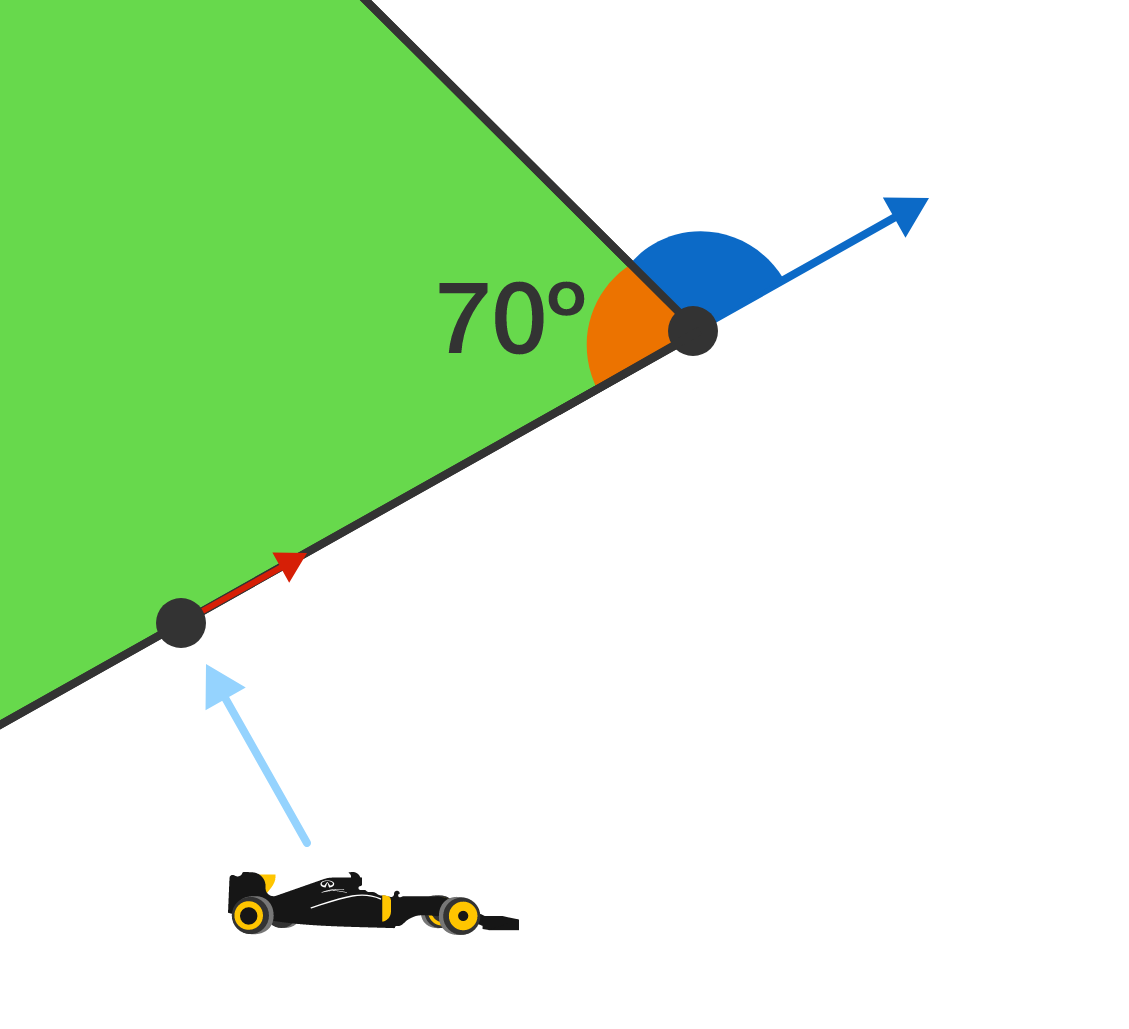Anna the racecar driver makes a left turn as shown. What is the measure of the blue angle (aka, how sharply does the car need to turn at that corner)?

# Driving on a Polygon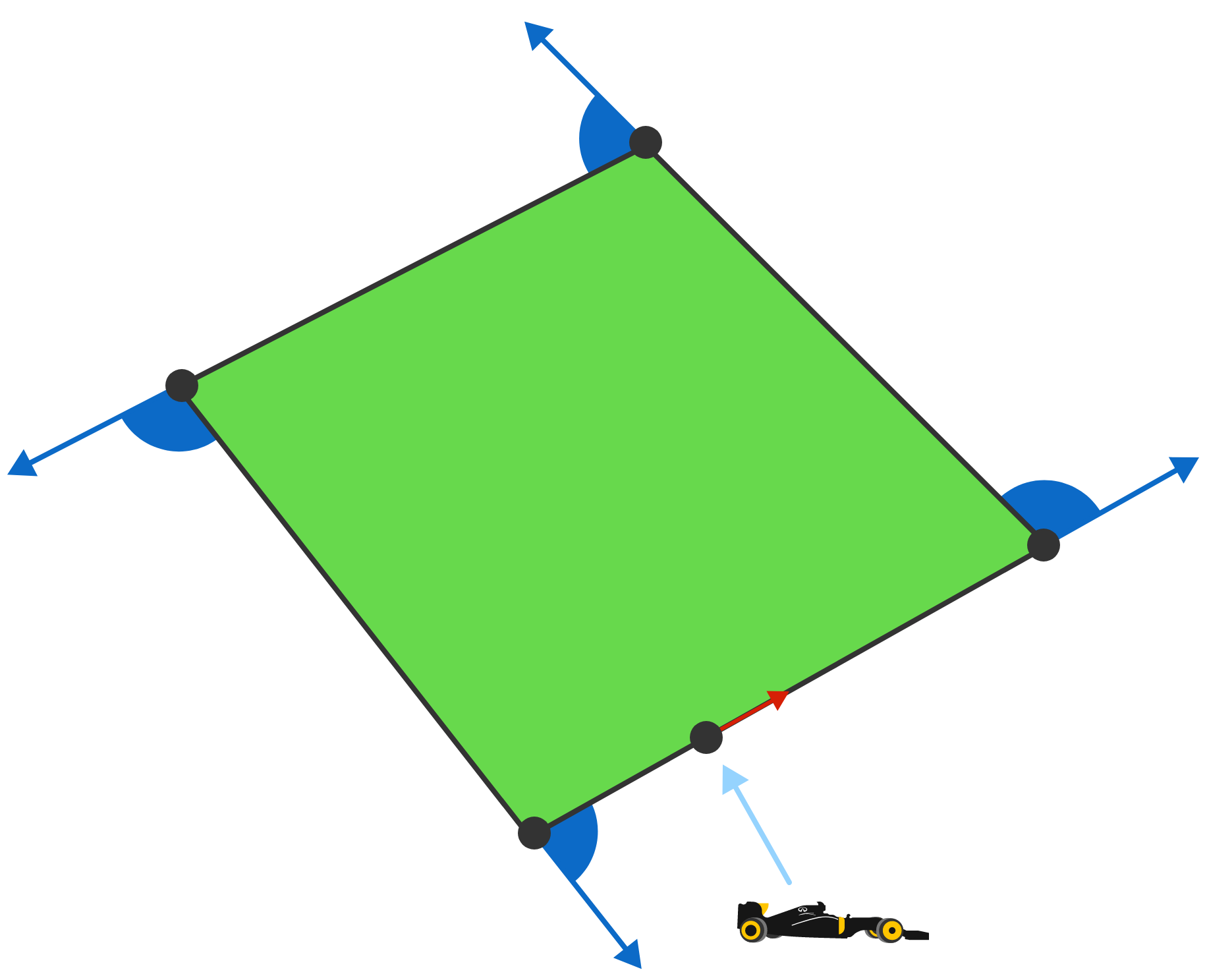Anna the racecar driver is on a flat track with four left turns, as shown above. The blue angles represent how much she turned at each corner.

What is the sum of all the blue angles?

# Driving on a Polygon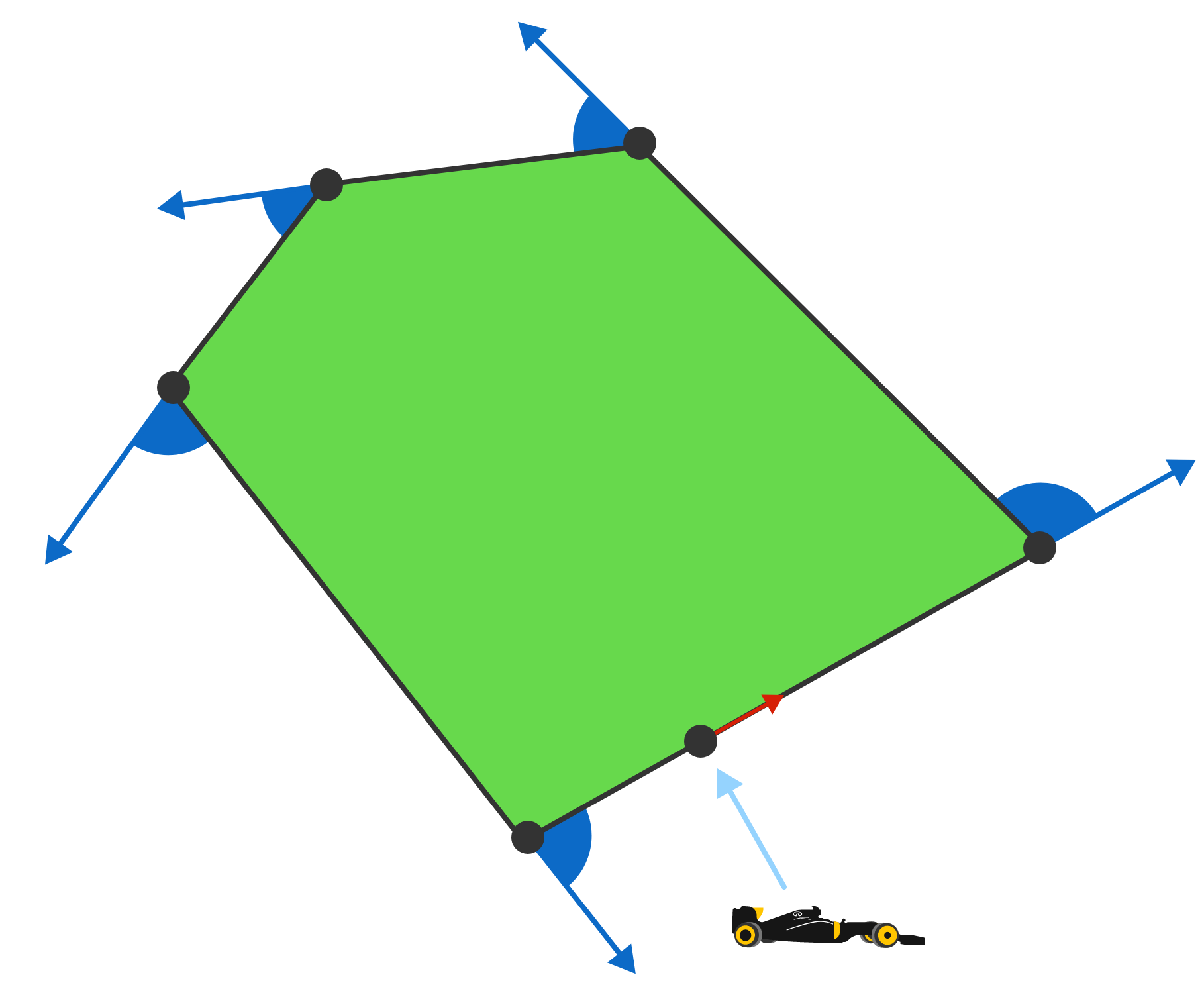Consider the same track but with one extra left turn added. Is the sum of blue angles (all 5 of them) now the same, less, or more than it was before?

# Driving on a Polygon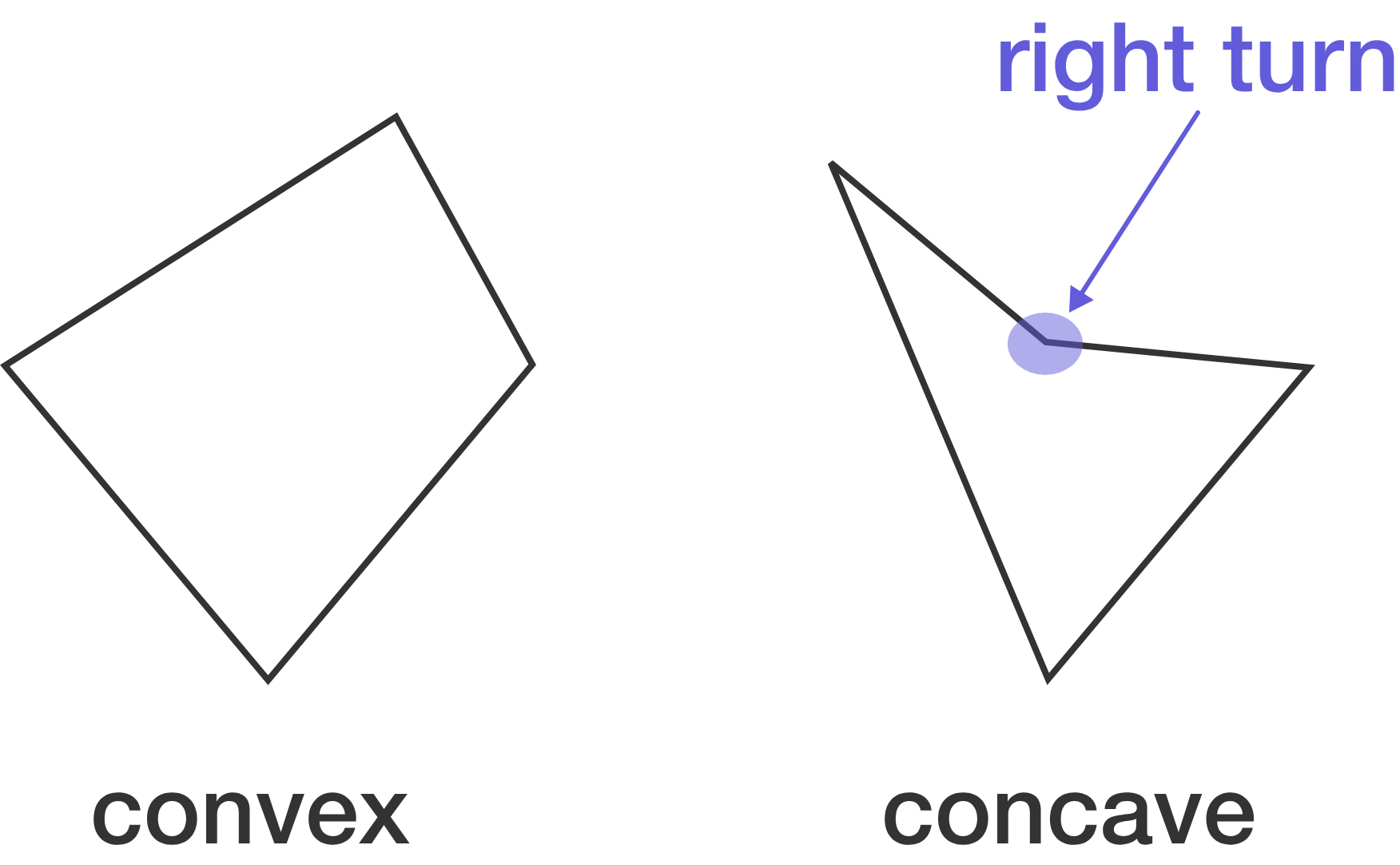The blue angles from the last two problems are the exterior angles of the polygon. Since we only had left turns, the polygon is called "convex":

The exterior angles of a convex polygon always add to $360^\circ .$

Since "it takes a full circle of turning to be facing back where you started" is an intuitive reason for the statement above to be true, we'll assume the statement is always true (that is, use it as an axiom).

By doing this, we'll guarantee our geometry is on a flat plane.

# Driving on a Polygon

• The exterior angles of a convex polygon always add to $360^\circ .$

• The angles that make up a straight line add to $180^\circ .$

From the axioms above, we can get how many degrees are in any polygon. Let's just start with a triangle: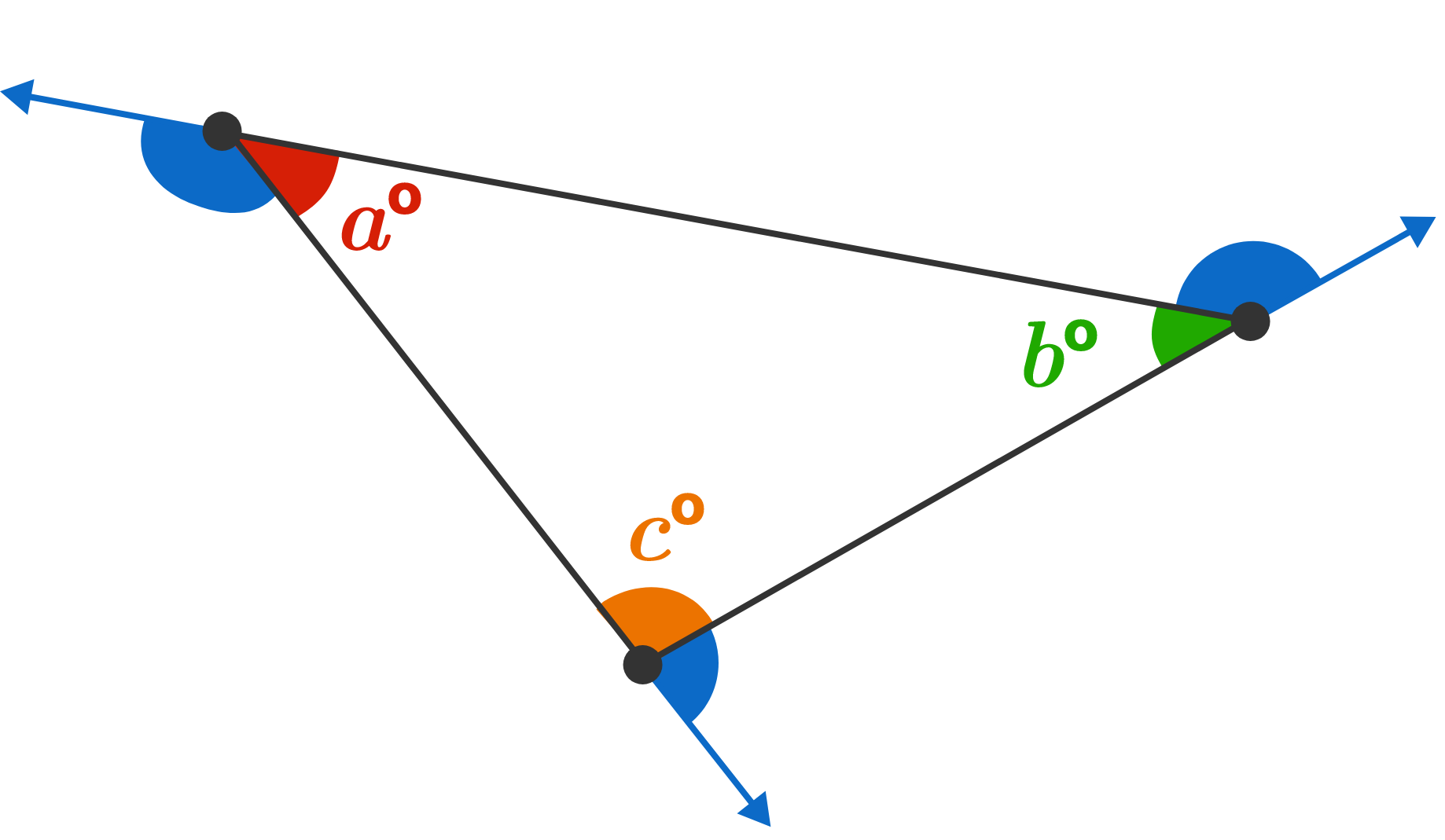$a + b + c$ is the sum of the interior angles of the polygon. Notice that we can combine the blue exterior angles with the interior angles and get three straight lines.

Based on the above, which equation is true?

# Driving on a PolygonGiven the result from the previous question $180 \times 3 = 360 + a + b + c,$ what must be true?

# Driving on a Polygon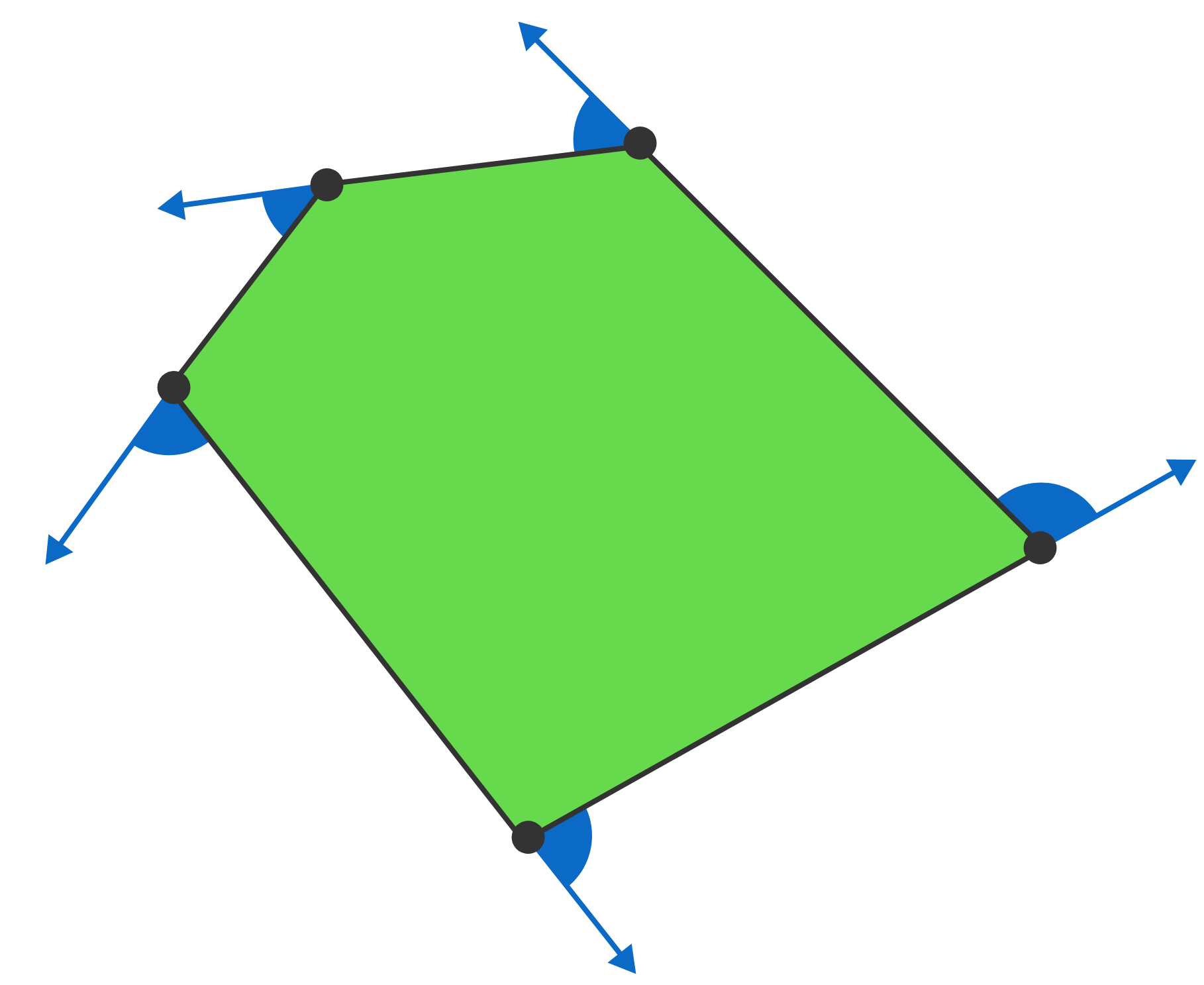Let's go back to one of the tracks earlier in the problem: what is the sum of interior angles of the polygon above?

# Driving on a Polygon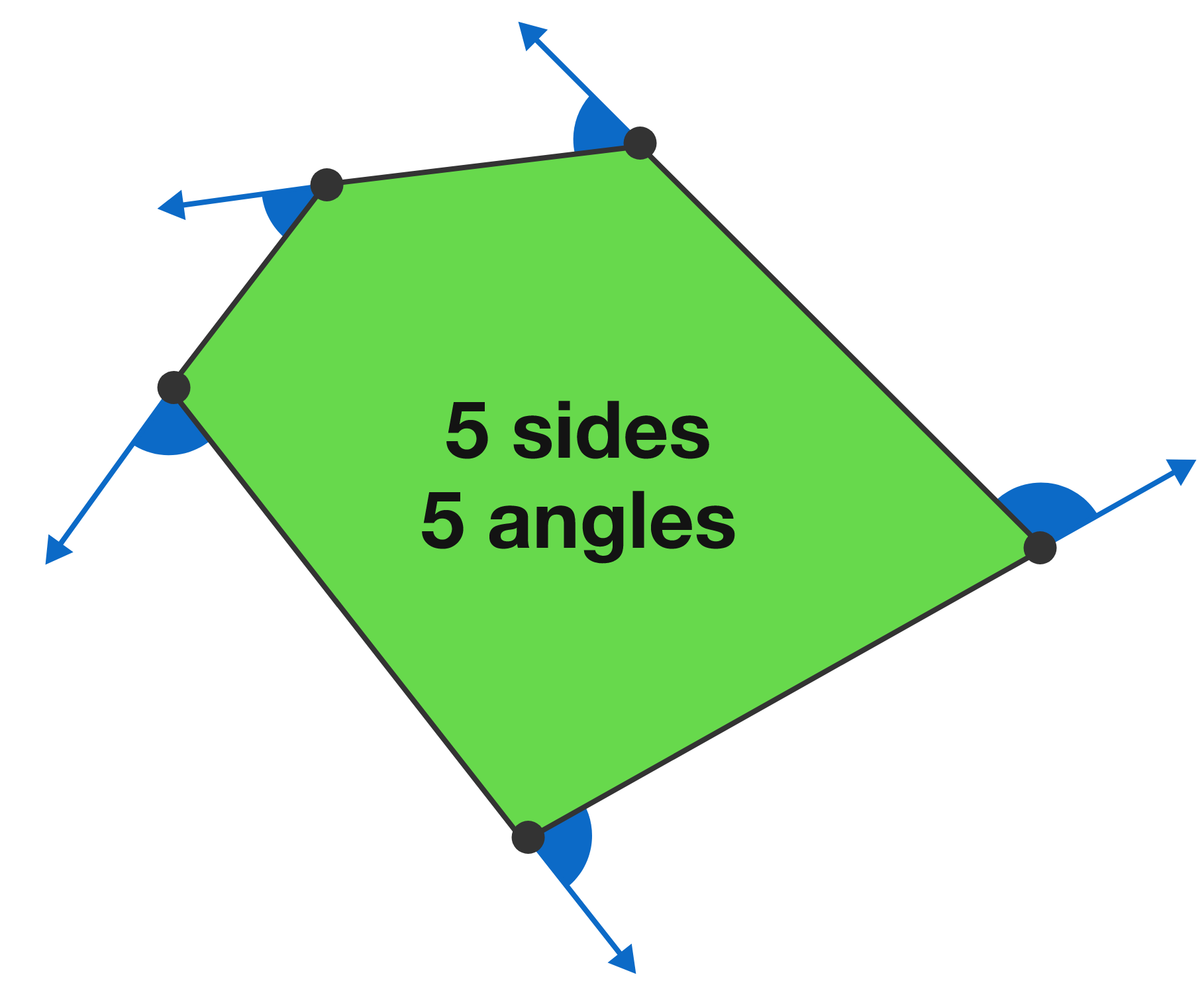In general, when dealing with convex polygons, the number of sides is the same as the number of straight lines formed by combining external and internal angles. So, while with ${\color{#D61F06}5}$ sides (and straight lines) we had ${\color{#D61F06}5} \times 180 = 360 + (\text{sum of interior angles}),$ we can generalize for ${\color{#D61F06}n}$ sides and ${\color{#D61F06}n}$ straight lines with which formula?

A. $\ {\color{#D61F06}n} \times 360 = 180 + (\text{sum of interior angles})$

B. $\ {\color{#D61F06}n} \times 180 = 180 + (\text{sum of interior angles})$

C. $\ {\color{#D61F06}n} \times 180 = 360 + (\text{sum of interior angles})$

# Driving on a Polygon

Congratulations! You've just proven a major theorem in geometry. Given an $n$-sided convex polygon, $n \times 180 = 360 + (\text{sum of interior angles}).$ So on a 100-sided convex polygon, for instance, the sum of interior angles is just $(100 \times 180) - 360 = 17640$ degrees.

What about concave polygons; does the same formula hold, and if so, why?Embark on answering this and many other questions, along with other dazzling geometry puzzles, in the main part of the course. Onward!

# Driving on a Polygon

×